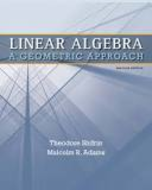Linear Algebra: A Geometric Approach - 2 Edition - Chapter 6.1 - Problem 15
Register Now

Join StudySoup

Get Full Access to Linear Algebra: A Geometric Approach - 2 Edition - Chapter 6.1 - Problem 159781429215213

# a. Let V = C1(I) be the vector space of continuously differentiable functions on the

Linear Algebra: A Geometric Approach | 2nd Edition

Problem 15

a. Let V = C1(I) be the vector space of continuously differentiable functions on the open interval I = (0, 1). Define T : V V by T (f )(t) = tf _ (t). Prove that every real number is an eigenvalue of T and find the corresponding eigenvectors. b. Let V = {f C0(R) : limt f (t)|t |n = 0 for all positive integers n}. (Why is V a vector space?) Define T : V V by T (f )(t) = _ t f (s)ds. (If f V , why is T (f) V ?) Find the eigenvalues and eigenvectors of T .

Accepted Solution
Step-by-Step Solution:

Step 1 of 2

The given vectors areand.

Find the dot product of both the given vectors.###### Chapter 6.1, Problem 15 is Solved

Step 2 of 2

Unlock Textbook Solution